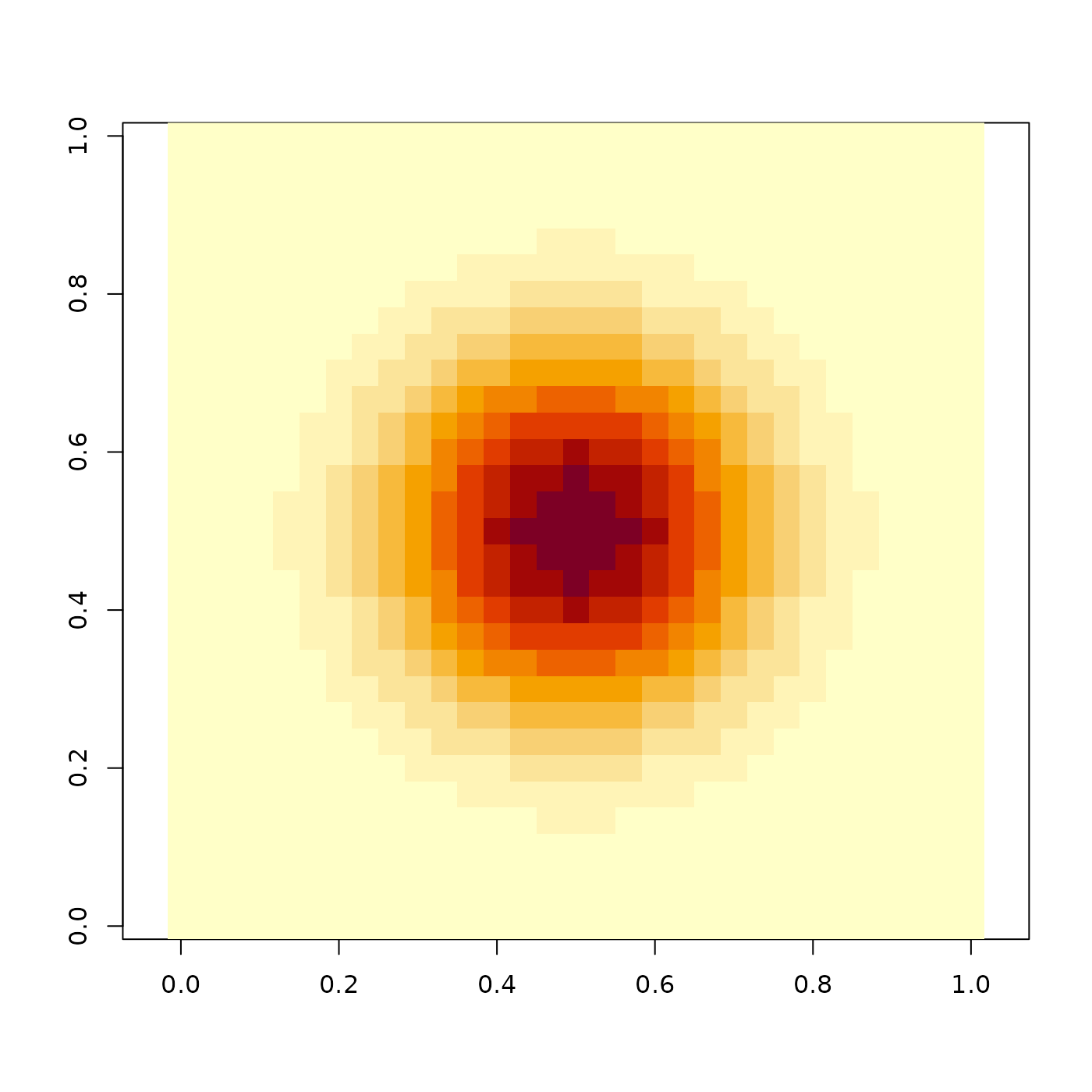Generates a 2D gaussian distribution, with an optional argument to take the gaussian to a user-defined power.

generate_2d_gaussian(
sd = 1,
power = 1,
dim = c(11, 11),
width = 3,
rescale_unity = FALSE
)

## Arguments

sd

Default 1. Standard deviation of the normal distribution

power

Default 1. Power to take the distribution. Higher values will result in a sharper peak.

dim

Default c(11, 11). The dimensions of the matrix.

width

Default 3 (-10 to 10). The range in which to compute the distribution.

rescale_unity

Default FALSE. If TRUE, this will rescale the max value to one. Useful if wanting to plot the distribution with plot_image().

## Examples

if(rayimage:::run_documentation()){
image(generate_2d_gaussian(1,1,31), asp=1)
}